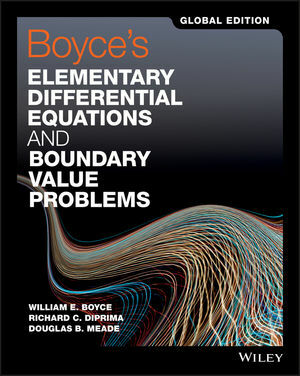# Elementary Differential Equations and Boundary Value Problems 11TH GE William Boyce / Richard Diprima 9781119382874

RM 150.00

TITLE :Boyce's Elementary Differential Equations and Boundary Value Problems 11TH GE William Boyce / Richard Diprima / Douglas Meade

ISBN13 : 9781119382874

PUBLISHER : JOHN WILEY (2017)

EDITION : 11TH GLOBAL EDITION PAPERBACK

PAGES : 624 Color Pages

## DESCRIPTION

Boyce's Elementary Differential Equations and Boundary Value Problems, like its predecessors, is written from the viewpoint of the applied mathematician, whose interest in differential equations may sometimes be quite theoretical, sometimes intensely practical, and often somewhere in between. The authors have sought to combine a sound and accurate (but not abstract) exposition of the elementary theory of differential equations with considerable material on methods of solution, analysis, and approximation that have proved useful in a wide variety of applications. While the general structure of the book remains unchanged, some notable changes have been made to improve the clarity and readability of basic material about differential equations and their applications. In addition to expanded explanations, this edition includes new problems, updated figures and examples to help motivate students.

The program is primarily intended for undergraduate students of mathematics, science, or engineering, who typically take a course on differential equations during their first or second year of study. The main prerequisite for engaging with the program is a working knowledge of calculus, gained from a normal two or three semester course sequence or its equivalent. Some familiarity with matrices will also be helpful in the chapters on systems of differential equations.

PREFACE vii

1 Introduction 1

2 First-Order Differential Equations 24

3 Second-Order Linear Differential Equations 101

4 Higher-Order Linear Differential Equations 167

5 Series Solutions of Second-Order Linear Equations 187

6 The Laplace Transform 239

7 Systems of First-Order Linear Equations 279

8 Numerical Methods 352

9 Nonlinear Differential Equations and Stability 386

10 Partial Differential Equations and Fourier Series 461

11 Boundary Value Problems and Sturm-Liouville Theory 527